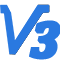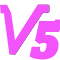Abstract
This paper presents an algorithm that utilizes the Hilbert curve for image encryption. The Hilbert curve is a space-filling curve known for its favorable spatial filling properties and one-dimensional characteristics. The proposed algorithm achieves image encryption and restoration by rearranging the pixels of the image in the order dictated by the Hilbert curve.

Hilbert curveimage encryptionone-dimensionalinformation security

1.希尔伯特曲线及其特性

import numpy as np
from hilbert import decode
import matplotlib.pyplot as plt

def hilbert_curve_points(order):
# 计算希尔伯特曲线的点数
num_points = 2 ** (order * 2)

# 生成希尔伯特曲线上所有点的 Hilbert 编码
curve_points = np.arange(num_points)

# 将 Hilbert 编码转换为坐标位置
coords = decode(curve_points, 2, order)

# 将坐标位置转换为一系列坐标
curve_coords = [(x, y) for x, y in coords]

return curve_coords

# 定义希尔伯特曲线的阶数
order = 3

# 生成阶数为3的希尔伯特曲线上所有点的坐标
curve_points = hilbert_curve_points(order)

# 提取 x 和 y 坐标
x_coords, y_coords = zip(*curve_points)

# 绘制希尔伯特曲线
plt.plot(x_coords, y_coords, marker='o', linestyle='-')
plt.title(f"Hilbert Curve of Order {order}")
plt.xlabel("X")
plt.ylabel("Y")
plt.grid(True)
plt.show()

2.利用希尔伯特曲线加解密图片

3.算法的用途

import cv2
import numpy as np
import matplotlib.pyplot as plt
from hilbert import decode

def hilbert_curve_points(order):
# 计算希尔伯特曲线的点数
num_points = 2 ** (order * 2)

# 生成希尔伯特曲线上所有点的 Hilbert 编码
curve_points = np.arange(num_points)

# 将 Hilbert 编码转换为坐标位置
coords = decode(curve_points, 2, order)

# 将坐标位置转换为一系列坐标
curve_coords = [(x, y) for x, y in coords]

return curve_coords

def shift_channel(channel, offset):
shifted_channel = np.roll(channel, -offset)
return shifted_channel

def shift_channels(pixels, num_channels, offset):
shifted_pixels = np.zeros_like(pixels)
for i in range(num_channels):
shifted_pixels[:, i] = shift_channel(pixels[:, i], offset)
return shifted_pixels

def Hilbert_encode(img, step):
# 获取图像的大小和通道数
height, width, channels = img.shape

# 计算希尔伯特曲线的级别
level = int(np.log2(max(height, width)))

# 生成希尔伯特曲线上的坐标点
curve_coords = hilbert_curve_points(level)

# 创建一个空的一维数组来存储新的像素值，对每个通道分别进行操作
pixels = np.zeros((height * width, channels), dtype=img.dtype)

# 使用希尔伯特曲线的顺序来获取像素值
for channel in range(channels):
for i, (x, y) in enumerate(curve_coords):
x = int(x)
y = int(y)
pixels[i, channel] = img[y % height, x % width, channel]

pixels = shift_channels(pixels, 3, step)

# 创建一个空的数组来存储还原的像素值
restored_img = np.zeros((height, width, channels), dtype=img.dtype)

# 使用希尔伯特曲线的坐标来填充像素值
for i, (x, y) in enumerate(curve_coords):
x = int(x)
y = int(y)
for channel in range(channels):
restored_img[y % height, x % width, channel] = pixels[i, channel]
return restored_img

# 读取图像

restored_img = Hilbert_encode(img, step=114514)

# 使用matplotlib显示还原的图像
plt.imshow(restored_img)
plt.axis('off')  # 隐藏坐标轴
plt.show()
# 保存处理后的图像
cv2.imwrite('out.jpg', restored_img)

12

13

{{notice.noticeContent}}
~~空空如也UICalc6个月16天前 修改于 6个月16天前 IP:上海
920647

lz是想用来直播吗？

12

##### 200字以内，仅用于支线交流，主线讨论请采用回复功能。6个月16天前 IP:安徽
920658

11

##### 200字以内，仅用于支线交流，主线讨论请采用回复功能。yuanfeng作者
6个月16天前 IP:湖南
920656

7

##### 200字以内，仅用于支线交流，主线讨论请采用回复功能。6个月16天前 IP:广东
920645

4

##### 200字以内，仅用于支线交流，主线讨论请采用回复功能。UICalc6个月16天前 修改于 6个月16天前 IP:上海
920647

lz是想用来直播吗？

12

##### 200字以内，仅用于支线交流，主线讨论请采用回复功能。yuanfeng作者
6个月16天前 IP:湖南
920654

4

##### 200字以内，仅用于支线交流，主线讨论请采用回复功能。20！Dopaminor6个月16天前 IP:广东
920655

3

##### 200字以内，仅用于支线交流，主线讨论请采用回复功能。yuanfeng作者
6个月16天前 IP:湖南
920656

7

##### 200字以内，仅用于支线交流，主线讨论请采用回复功能。6个月16天前 IP:安徽
920658

11

##### 200字以内，仅用于支线交流，主线讨论请采用回复功能。6个月10天前 IP:江西
920850

2

##### 200字以内，仅用于支线交流，主线讨论请采用回复功能。5个月18天前 IP:广东
921805

##### 200字以内，仅用于支线交流，主线讨论请采用回复功能。4个月16天前 IP:山东
922780

3

##### 200字以内，仅用于支线交流，主线讨论请采用回复功能。2个月28天前 IP:广东
924979

##### 200字以内，仅用于支线交流，主线讨论请采用回复功能。20！Dopaminor2个月3天前 IP:广东
925633

##### 200字以内，仅用于支线交流，主线讨论请采用回复功能。1个月20天前 IP:广东
926121

##### 200字以内，仅用于支线交流，主线讨论请采用回复功能。yuanfeng进士 机友 笔友

7

92

0
2019/07/21注册，21天19时前活动

IP归属地：湖南

{{errorInfo}}

##### 当前账号的附件下载数量限制如下：

{{f.startingTime}}点 - {{f.endTime}}点 {{f.fileCount}}

{{tip}}
{{reason.type}}

#### 空空如也

{{format('YYYY/MM/DD HH:mm:ss', toc)}}